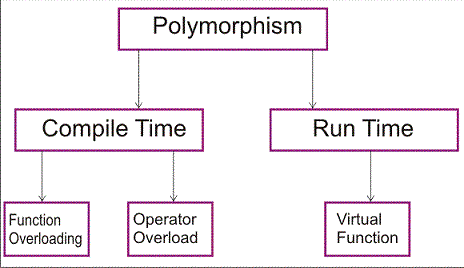# C++ language

Polymorphism
 Previous Home Next

Polymorphism means one thing in many form. In polymorphism operators and functions can use in many forms. Single operator behave different for different data type. Such as addition operator use to add integer and concatenate for string.

Polymorphism is the concept where one same function or operator send to many different object how perform different operation and result is also different.

### Types of polymorphism

1. Static Polymorphism
2. Dynamic PolymorphismStatic polymorphism: refers to an many similar entity existing in continuous but have slight difference. Static polymorphism many functions have some differences as number, type, and sequence of arguments. When function declaration the various types of parameters are specified,

and therefore the function can be bound to calls at compile time. This phenomena is called early binding. The term early binding the calls of parameter , return type are already bound to that functions when program is executed. At the time of compilation this function bind that's why its known as early binding.

### Function Overloading:

In function overloading provide the facility that with in the same class more then one function can have same name but the number , type and appearance of the argument or parameter must be changed.

How to declare Function in function overloading:

```void fun();	// Overloaded
void fun(int i,
float j); // Overloaded
void fun(float
j,inti);  // Overloaded
void fun(int i,
int j); // Overloaded
void fun(int j,
int i); // Overloaded
int fun();
//Error - function redefined
(because only return type is different)
```

### Example

```#include <iostream.h>
class Maths
{
public:
int volume(int i)
{
return(i*i);
}
double volume(double j,
int k)
{
return(j*k);
}
long volume(long l,
int x,int y)
{
return(l*x*y);
}
};
```

### Operator Overloading:

Operator overloading  concept where operator overloaded. In general operator will be applied on variables or on constant but in operator overloading operator use as the name of function and operator applied on object.

Types of operator who overload are:
• overload plus subtract
• overload object and primitive data type
• overload assignment operator
• overload logic operator
• overload conversion operator
• overload pointer operator
• overload address of operator
• overload unary operator
• overload bracket operator
• overload square bracket
• overload cast operator
• overload comma operator
• overload new operator
• overload delete operator
• overload ostream istream operator
• overload operator with friend
• overload with friend function
• overloading Dereference operator
• custom extractor
• custom inserter
• enum operator

### Example:

```#include <iostream>
using namespace std;

class Op_overload {
int x, y, z;
public:
Op_overload() { x = y = z = 0; }
Op_overload(int i,
int j, int
k) { x = i; y = j; z = k; }

Op_overload operator+(Op_overload op2);
// op1 is implied
Op_overload operator=(Op_overload op2);
// op1 is implied
Op_overload operator-(Op_overload op2);
// op1 is implied
void show() ;
};
Op_overload Op_overload::operator-(Op_overload op2)
// subtraction overload .
{
Op_overloadtemp_store;

temp_store.x = x - op2.x;
temp_store.y = y - op2.y;
temp_store.z = z - op2.z;
returntemp_store;
}

Op_overload Op_overload::operator
+(Op_overload op2) // addition overload.
{
Op_overloadtemp_store;

temp_store.x = x + op2.x;
temp_store.y = y + op2.x;
temp_store.z = z + op2.z;
returntemp_store;
}
Op_overload Op_overload::operator
=(Op_overload op2)  // assignment overload .
{
x = op2.x;
y = op2.y;
z = op2.z;
return *this;
}

// Show X, Y, Z coordinates.
void Op_overload::show()
{
cout << x <<
", ";
cout << y <<
", ";
cout << z <<
"\n";
}

int main()
{
Op_overload a(10,
20, 30)
, b(100,
100, 100), c;

cout << "value of a: ";
a.show();
cout << "value of b: ";
b.show();

c = a - c;
cout << "a - c: ";
c.show();

cout << "\n";

return 0;
}
```

Output:

```value of a: 10, 20, 30
value of b: 100, 100, 100
a - c: 10, 20, 30
```

Dynamic polymorphism where an entity can modify its form depending on the situation. A function is dynamic polymorphism when one function can have more then one form and the call of various form can be resolved at run time dynamically or at the time of execution.The termlate binding refers to the functions resolution at run-time . Late binding give more the flexibility of the program.

### Virtual Function:

A function which become virtual function then use virtual keyword. a virtual function is a member function which is defined in base class and then redefine for other derived classes,

and the redefined virtual function will always called by the compiler for an object of the corresponding derived class, even if called by pointer or reference to a base class of the object to that function.

A class that declares or inherits a virtual function is called a polymorphic class.

### Example

```#include <iostream>
using namespace std;

class base
{
public:
void display()
{
cout<<"\n Display base "
;
}
virtual show()
{
cout<<"\n show base "
;
}

};
class derived:public base
{
public:
void display()
{
cout<<
"\n Display derived ";
}
void show()
{
cout<<
"\n show derived ";
}
};
int main()
{
base b;
derived d;
base *bptr;
cout<<
"\n bptr points to base\n";
bptr=&b;
bptr->display();  //calls base display
bptr->shoe();   //calls base show
cout<<"\n bptr points to derived
\n";
bptr=&d;
bptr->display();   // calls base display
bptr->show();    //calls derived show is virtual
return 0;
}
```

Output:

```bptr points to base

Display base
show Base
bptr points to derived

Display base
show derived
```
 Previous Home Next